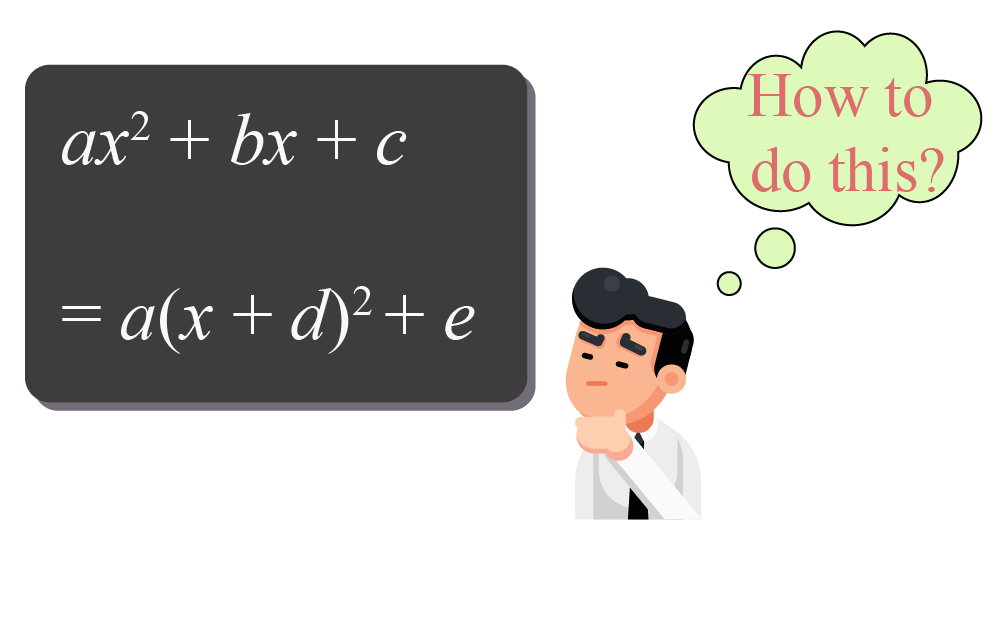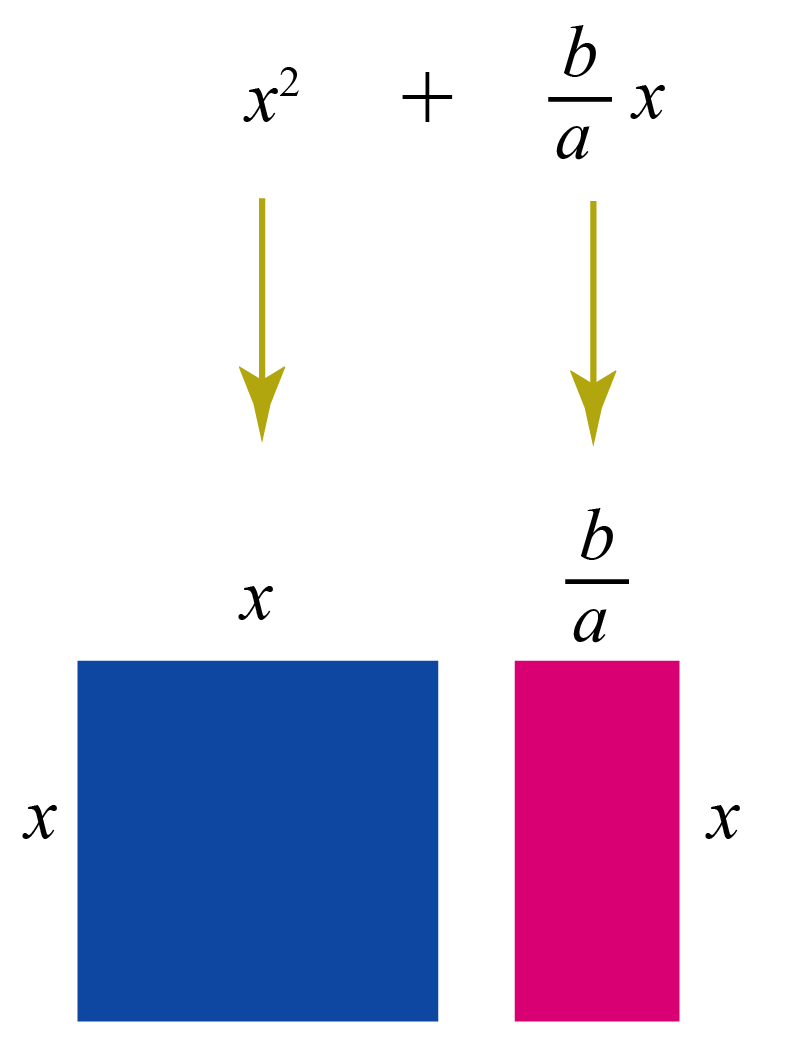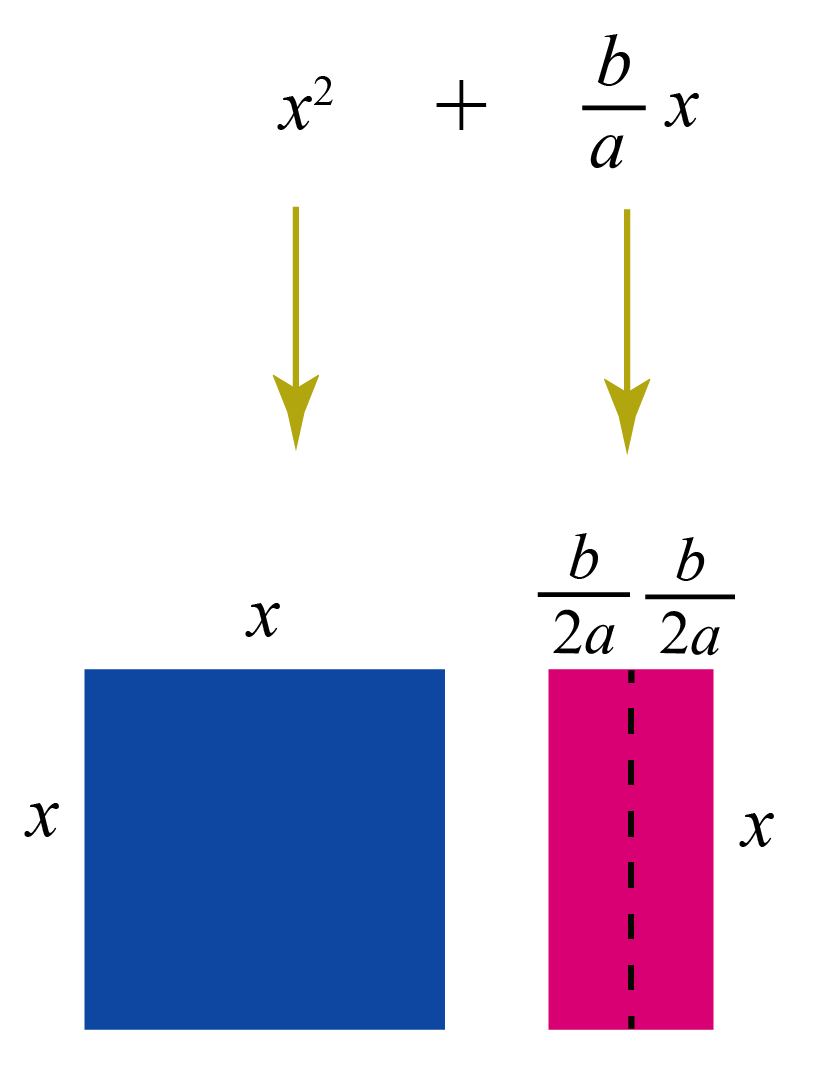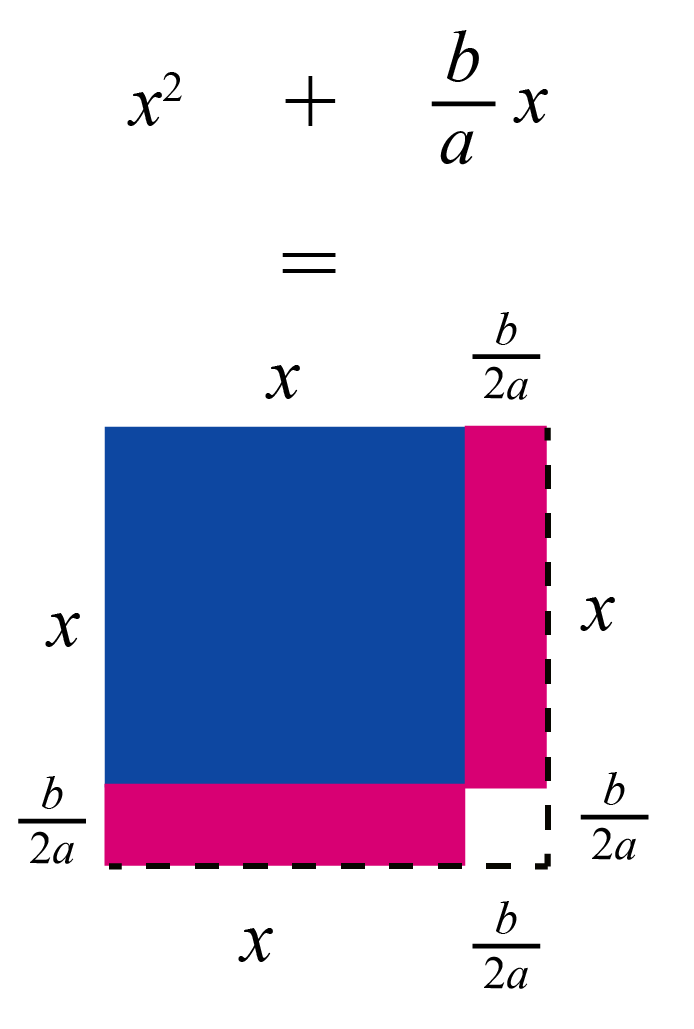# Completing the Square

Completing the Square

In this chapter, we will learn how to compete the squares of certain numbers. We will also learn completing the square formula,  have a look at completing the square examples, and the steps required in completing the squares. Check-out the interactive simulations to know more about the lesson and try your hand at solving a few interesting practice questions at the end of the page.

Completing the square is converting a quadratic expression of the form $$ax^2 + bx +c$$ to the vertex form  $$a(x+d) 2 + e$$

Completing the square is useful in:

• Converting a quadratic expression into vertex form
• Analyzing at which point the quadratic expression has minimum/maximum value

## Lesson Plan

 1 How to Complete the Square? 2 Tips and Tricks 3 Solved Examples on Completing the Square 4 Interactive Questions on Completing the Square 5 Challenging Questions on Completing the Square

## How to Complete the Square

We know that a quadratic equation of the form $$ax^2+bx+c=0$$ can be solved by the factorization method.

But sometimes, factorizing the quadratic expression $$ax^2+bx+c$$ is NOT possible.

For example

$$x^2 +2x+3$$ cannot be factorized as we cannot find two numbers whose sum is $$2$$ and whose product is $$3$$

In such cases, we write it in the form $$a(x+d)^2+e$$ by completing the square.

Since we have $$(x+d)$$ whole squared, we say that we have "completed the square" here.

But, how do we complete the square?## Steps to Complete the Square

Let us learn how to complete a square using an example.

Example

Complete the square in the expression

$-4 x^{2}-8 x-12$

Solution:

First, we should make sure that the coefficient of $$x^2$$ is $$1$$

If the coefficient of $$x^2$$ is NOT $$1$$, we will place the number outside as a common factor.

We will get:

$-4 x^{2}-8 x-12 = -4 (x^2+2x+3)$

Now, the coefficient of $$x^2$$ is $$1$$

Step 1: Find half of the coefficient of $$x$$

Here, the coefficient of $$x$$ is $$2$$

Half of $$2$$ is $$1$$

Step 2: Find the square of the above number

$1^2=1$

Step 3: Add and subtract the above number after the $$x$$ term in the expression whose coefficient of $$x^2$$ is $$1$$

\begin{align} -4 (x^2\!+\!2x\!+\!3)\!&=\!\!-4 \left(x^2\!+\!2x\! +\color{green}{\mathbf{1 -1}} \!+\!3 \right)\end{align}

Step 4: Factorize the perfect square trinomial formed by the first 3 terms using the identity $$x^2+2xy+y^2=(x+y)^2$$

In this case, $x^2+2x+ 1= (x+1)^2$

The above expression from Step 3 becomes:

$-4 \left(\color{green}{x^2\!+\!2x \!+\!1\!}-\!1 \!+3\right) = -4 (\!\color{green}{(x+1)^2}\!\! -\!1+3\!)$

Step 5: Simplify the last two numbers.

Here, $$-1+3=2$$

Thus, the above expression is:

$-4 (x+1)^2 \color{green}{-1+3} = -4 ((x+1)^2 +\color{green}{2}) = \\-4(x+1)^2-8$

This is of the form $$a(x+d)^2+e$$.

Hence, we have completed the square.

 Thus, $$-4 x^2-8 x-12= -4 (x+1)^2 -8)$$

You can find more examples of completing the square in the "Solved Examples" section of this page.

## Complete the Square Calculator

We can enter any quadratic expression here and see how the square can be completed.Tips and Tricks

To complete the square in an expression
$ax^2+bx+c$

• Make sure the coefficient of $$x^2$$ is $$1$$
• Add and subtract $$\left( \dfrac{-b}{2a}\right)^2$$ after the $$x$$ term and simplify

## Formula of Completing the Square

Instead of using complex methods such as "complete the square" and "complete the square using Geometry," we can use the following simple formula to complete the square.

To complete the square in the expression $$ax^2+bx+c$$, first find:

 \begin{align} d &=\dfrac{b}{2a} \end{align}  and  \begin{align} e=c- \dfrac{b^2}{4a} \end{align}

Substitute these values in:

 $$a x^2+b x+c=a(x+d)^2+e$$

These formulas are derived geometrically. Are you curious to know how?

Then you can click on the "+" sign against the following.

It also includes an illustration where you can clearly understand how to complete the square geometrically.

## Completing the Square Using Geometry (with Illustration)

Let us complete the square in the expression $$ax^2+bx+c$$ using Geometry.

Based on the method studied earlier, the coefficient of $$x^2$$ must be made $$1$$ by taking $$a$$ as the common factor.

We get, $ax^2\!+\!bx\!+\!c\!=\!a \left(\!\!x^2+\! \dfrac{b}{a}x+ \!\dfrac{c}{a} \!\!\right) \!\!\rightarrow\!\! (1)$

Now, we will consider the first two terms, $$x^2$$ and $$\dfrac{b}{a}x$$

Let us consider a square of side $$x$$ (whose area is $$x^2$$)

Let us also consider a rectangle of length $$\dfrac{b}{a}$$ and breadth $$x$$ (whose area is $$\dfrac{b}{a}x$$)Now, divide the rectangle into two equal parts.

The length of each rectangle will be $$\dfrac{b}{2a}$$Attach half of this rectangle to the right side of the square and the remaining half to the bottom of the square.To complete a geometric square, there is some shortage which is a square of side $$\dfrac{b}{2a}$$

The square of area $$\left( \dfrac{b}{2a}\right)^2$$ should be added to $$x^{2}+\dfrac{b}{a} x$$ to complete the square.

You can visualize the same thing using the following illustration.

But, we cannot just add, we need to subtract it as well to retain the expression's value.

Thus, to complete the square:

\begin{aligned}x^2+ \dfrac{b}{a}x &= x^2+ \dfrac{b}{a}x + \left( \dfrac{b}{2a}\right)^2- \left( \dfrac{b}{2a}\right)^2 \0.2cm] &= x^2+ \dfrac{b}{a}x + \left( \dfrac{b}{2a}\right)^2 - \dfrac{b^2}{4a^2}\\[0.2cm]& \text{Multiplying and dividing }\dfrac{b}{a}x \text{ with 2}\\[0.2cm] &= x^2+ 2 \cdot x \cdot \dfrac{b}{2a} + \left( \dfrac{b}{2a}\right)^2 - \dfrac{b^2}{4a^2}\\[0.2cm] \end{aligned} By using the identity \[x^2+2xy+y^2=(x+y)^2

the above equation can be written as,

$x^2+ \dfrac{b}{a}x = \left(x + \dfrac{b}{2a}\right)^2- \dfrac{b^2}{4a^2}$

By substituting this in (1):

\begin{aligned}ax^2+bx+c&=a \left(\!\! \left(x + \dfrac{b}{2a}\right)^2\!- \dfrac{b^2}{4a^2}+ \dfrac{c}{a} \!\right)\0.2cm] &=a \left(x + \dfrac{b}{2a}\right)^2 - \dfrac{b^2}{4a}+ c \\[0.2cm]&=a \left(x + \dfrac{b}{2a}\right)^2 + \left(c- \dfrac{b^2}{4a} \right) \end{aligned} This is of the form $$a(x+d)^2+e$$ where \[ \begin{aligned} d &=\dfrac{b}{2a}\\[0.2cm] e &=c- \dfrac{b^2}{4a} \end{aligned}

Example:

We will complete the square in $$-4 x^{2}-8 x-12$$ (which is the same as the above example) using this formula.

Comparing this with $$ax^2+bx+c$$,

$a=-4\\b=-8\\c=-12$

Find the values of $$d$$ and $$e$$ using:

\begin{aligned} d &=\dfrac{b}{2a}= \dfrac{-8}{2(-4)}= 1\\[0.2cm] e &=c- \dfrac{b^2}{4a}= -12-\dfrac{(-8)^2}{4(-4)}= -8 \end{aligned}

Substitute these values in:

$a x^{2}+b x+c=a(x+d)^{2}+e$

 We get: $$-4 x^2-8 x-12= -4 (x+1)^2 -8$$

We can observe that we have arrived at the same answer by using the formula.

## Solved Examples

Here are some examples of completing the square.

 Example 1

What number should be added to $$x^2-7x$$ in order to make it a perfect square trinomial?

Solution:

The given expression is $$x^2-7x$$

Method 1:

The coefficient of $$x$$ is $$-7$$

Half of this number is $$\dfrac{-7}{2}$$

Finding the square,

$\left(\dfrac{-7}{2} \right)^2= \dfrac{49}{4}$

Method 2:

Comparing the given expression with $$ax^2+bx+c$$,

\begin{align}a&=1 \\ b&=-7 \end{align}

The term that should be added to make the given expression a perfect square trinomial is,

$\left( \dfrac{-b}{2a}\right)^2 = \left( \dfrac{-7}{2(1)} \right)^2= \dfrac{49}{4}$

Thus, from both the methods, the term that should be added to make the given expression a perfect square trinomial is,

 $$\therefore$$ $$\dfrac{49}{4}$$
 Example 2

Complete the square in the quadratic expression $$2 x^{2}+7 x+6$$.

Solution:

The given expression is $$2 x^{2}+7 x+6$$

To complete the square, first, we will make the coefficient of $$x^2$$ as $$1$$

We will take the coefficient of $$x^2$$ (which is $$2$$) as a common factor.

$2 x^{2}+7 x+6 = 2\left( x^2 + \dfrac{7}{2}x+ 3 \right) \,\,\,\,\,\rightarrow (1)$

The coefficient of $$x$$ is $$\dfrac{7}{2}$$

Half of it is $$\dfrac{7}{4}$$

Its square is $$\left( \dfrac{7}{4} \right)^2= \dfrac{49}{16}$$

This term can also be found using $$\left( \dfrac{-b}{2a}\right)^2 = \left( \dfrac{-7}{2(2)} \right)^2= \dfrac{49}{16}$$

Add and subtract it after the $$x$$ term in (1):

$2 x^{2}\!+\!7 x\!+\!6 = 2\left(\!\!x^2 \!+\! \dfrac{7}{2}x\!+\!\dfrac{49}{4}\!-\!\dfrac{49}{4} +3 \!\!\right)$

Factorize the trinomial made by the first three terms:

\begin{aligned}2 x^{2}\!+\!7 x\!+\!6 &= 2\left( \!x^2 + \dfrac{7}{2}x+\dfrac{49}{16}-\dfrac{49}{16}+3\! \right)\\[0.2cm] &= 2 \left(\!\! \left(x+ \dfrac{7}{4} \right)^2 -\dfrac{49}{16}+3 \right)\\ &= 2 \left( \left(x+ \dfrac{7}{4} \right)^2 -\dfrac{1}{16} \right)\\ &= 2\left(x+ \dfrac{7}{4} \right)^2 - \dfrac{1}{8} \end{aligned}

The final answer is of the form $$a(x+d)^2+e$$

Hence, the competing of square is done.

Thus,

 $$2 x^2+7 x+6= 2\left(x+ \dfrac{7}{4} \right)^2 - \dfrac{1}{8}$$
 Example 3

Solve by completing the square.

$x^2-10x+16=0$

Solution:

$x^2-10x+16=0$

We will solve by completing the square.

Here, the coefficient of $$x^2$$ is already $$1$$

The coefficient of $$x$$ is $$-10$$

The square of half of it is $$(-5)^2 =25$$

Adding and subtracting it on the left-hand side of the given equation after the $$x$$ term:

\begin{aligned} x^2-10x+25-25+16&=0\\[0.2cm](x-5)^2-25+16&=0\\ [\because x^2\!-\!10x\!+\!25\!=\! (x\!-\!5)^2 ]\\[0.2cm] (x-5)^2-9&=0\\[0.2cm] (x-5)^2& =9 \\[0.2cm] (x-5) &= \pm\sqrt{9} \\ [ \text{Taking square root }&\text{on both sides} ]\\[0.2cm] x-5=3; \,\,\,\,&x-5= -3\\[0.2cm] x=8; \,\,\,\,&x = 2 \end{aligned}

 $$\therefore$$ $$x=8,\, \, 2$$

## Interactive Questions

Here are a few problems for you to practice. Select/type your answer and click the "Check Answer" button to see the result.Challenging Questions
1. Solve by completing the square.
$x^4-18 x^2+17=0$
Hint: Assume $$x^2=t$$
2. Write the following equation of the form $$(x-h)^2+(y-k)^2=r^2$$ by completing the square.
$x^2+y^2-4 x-6 y+8=0$

## Let's Summarize

We hope you enjoyed learning about Completing the Square with the simulations and practice questions. Now you will be able to easily solve problems on completing the square, completing the square formula, completing the square examples, steps to completing the square.

At Cuemath, our team of math experts is dedicated to making learning fun for our favorite readers, the students!

Through an interactive and engaging learning-teaching-learning approach, the teachers explore all angles of a topic.

Be it worksheets, online classes, doubt sessions, or any other form of relation, it’s the logical thinking and smart learning approach that we at Cuemath believe in.

## 1. What number will complete the square?

$$\left( \dfrac{-b}{2a} \right)^2$$ will complete the square in the expression $$ax^2+bx$$,

For more information, you can go through the "Method of Completing the Square" and "Formula of Completing the Square" sections of this page.

## 2. How do you know when to complete the square?

We complete the square when the problem says "Solve by completing the square."

Also, when we are asked to convert a quadratic expression of the form $$ax^2+bx+c$$ to the vertex form $$a(x-h)^2+k$$, we complete the square.

## 3. How do you complete the square with two variables?

Let us see how to complete the square in the expression of two variables.

Consider an expression in two variables $$x^2+y^2+2x+4y+7$$

To complete the square, we just make each of the coefficients of $$x$$ and $$y$$ half and square it first.

$\left( \dfrac{2}{2}\right)^2=1\\[0.2cm] \left( \dfrac{4}{2}\right)^2=4$

Let us add and subtract this to the given equation.

Then rearrange the terms to complete the squares.

\begin{align} &x^2\!+\!y^2\!+\!2x\!+\!4y\!+\!7\!+\!(\!2-2\!)\!+\!(\!4-4\!)\\[0.2cm] &=(\!x^2\!+\!2x\!+\!1\!)\!\!+\!\!(\!y^2\!+\!4y\!+\!4\!)\!+\!7\!-\!2\!-\!4\\[0.2cm] &= (\!x+2\!)^2+(\!y+2\!)^2 +1 \end{align}

## 4. When should you complete the square?

We complete the square when the problem says "Solve by completing the square".

Also, when we are asked to convert a quadratic expression of the form $$ax^2+bx+c$$ to the vertex form $$a(x-h)^2+k$$, we complete the square.

More Important Topics
Numbers
Algebra
Geometry
Measurement
Money
Data
Trigonometry
Calculus